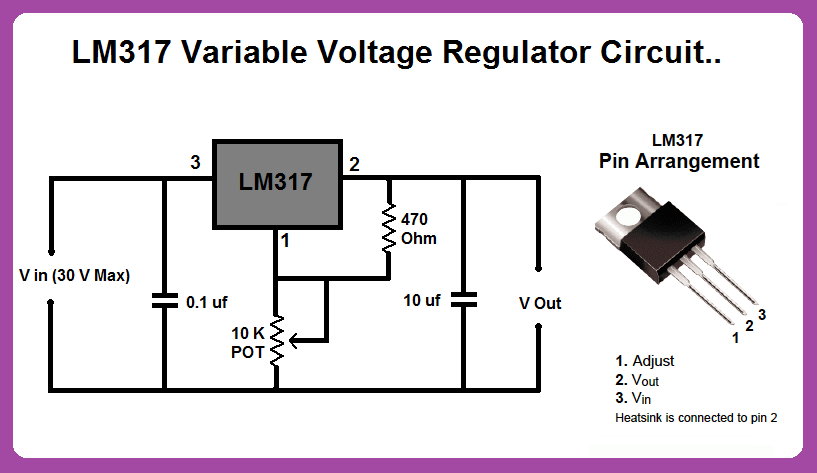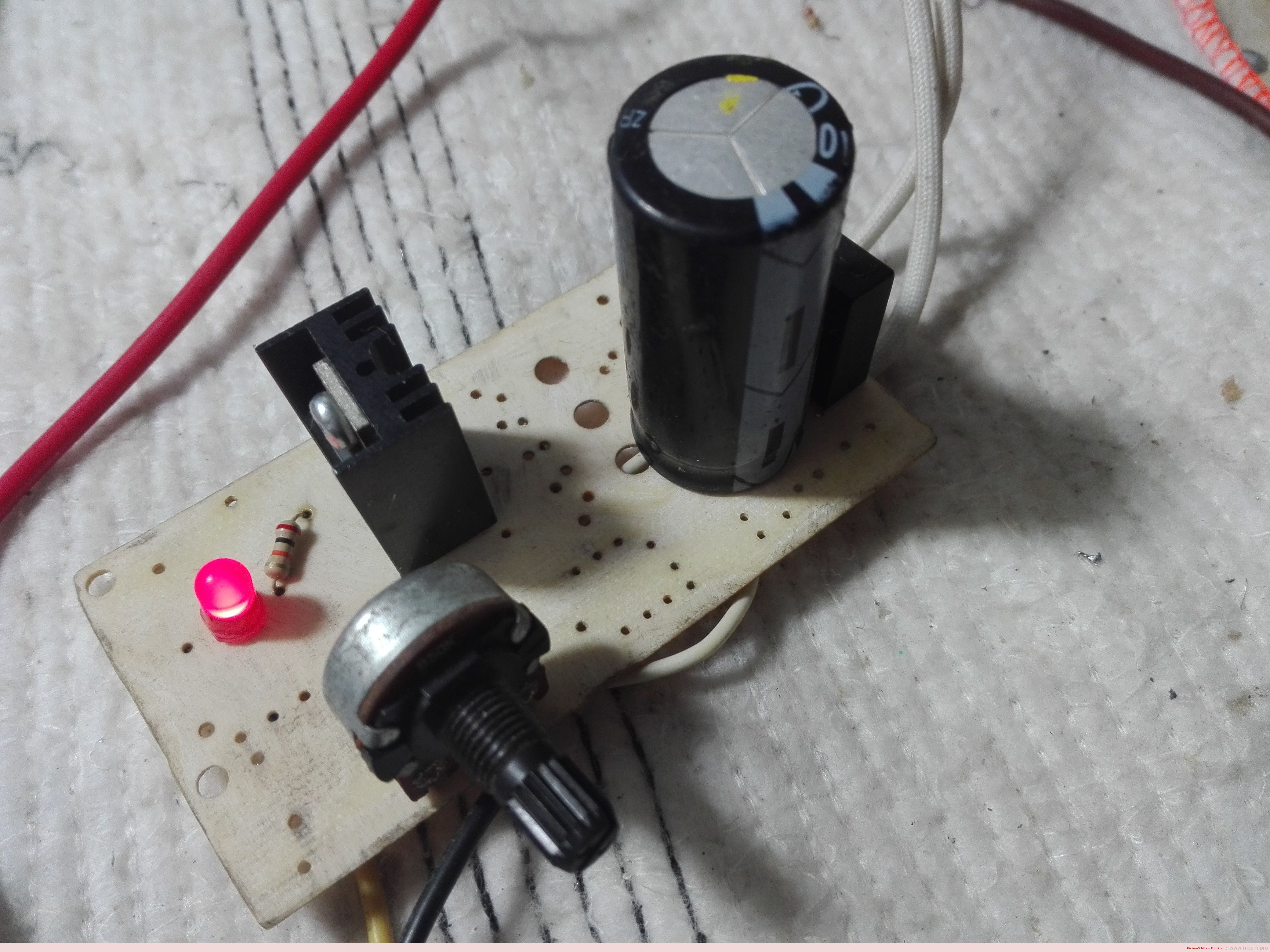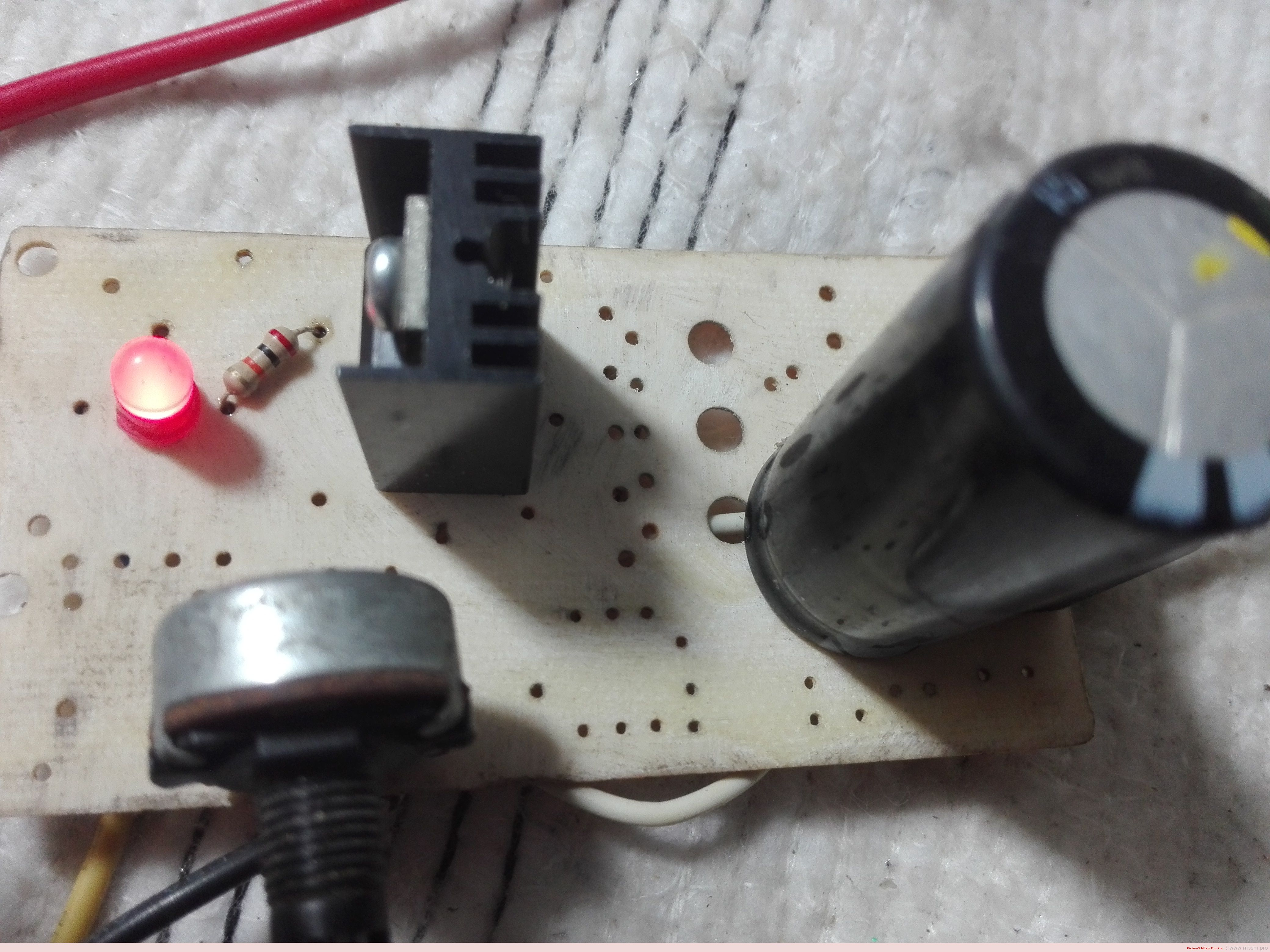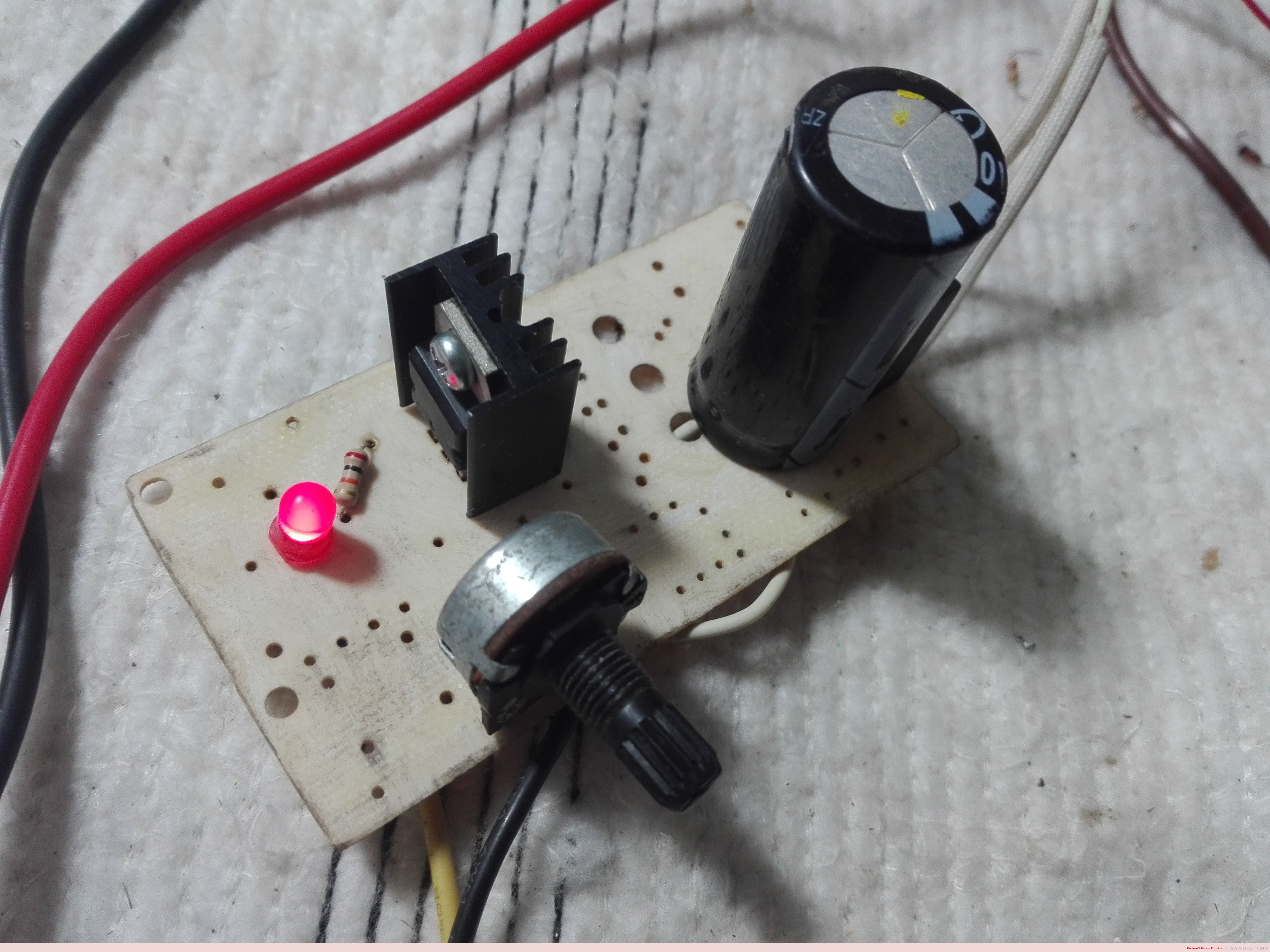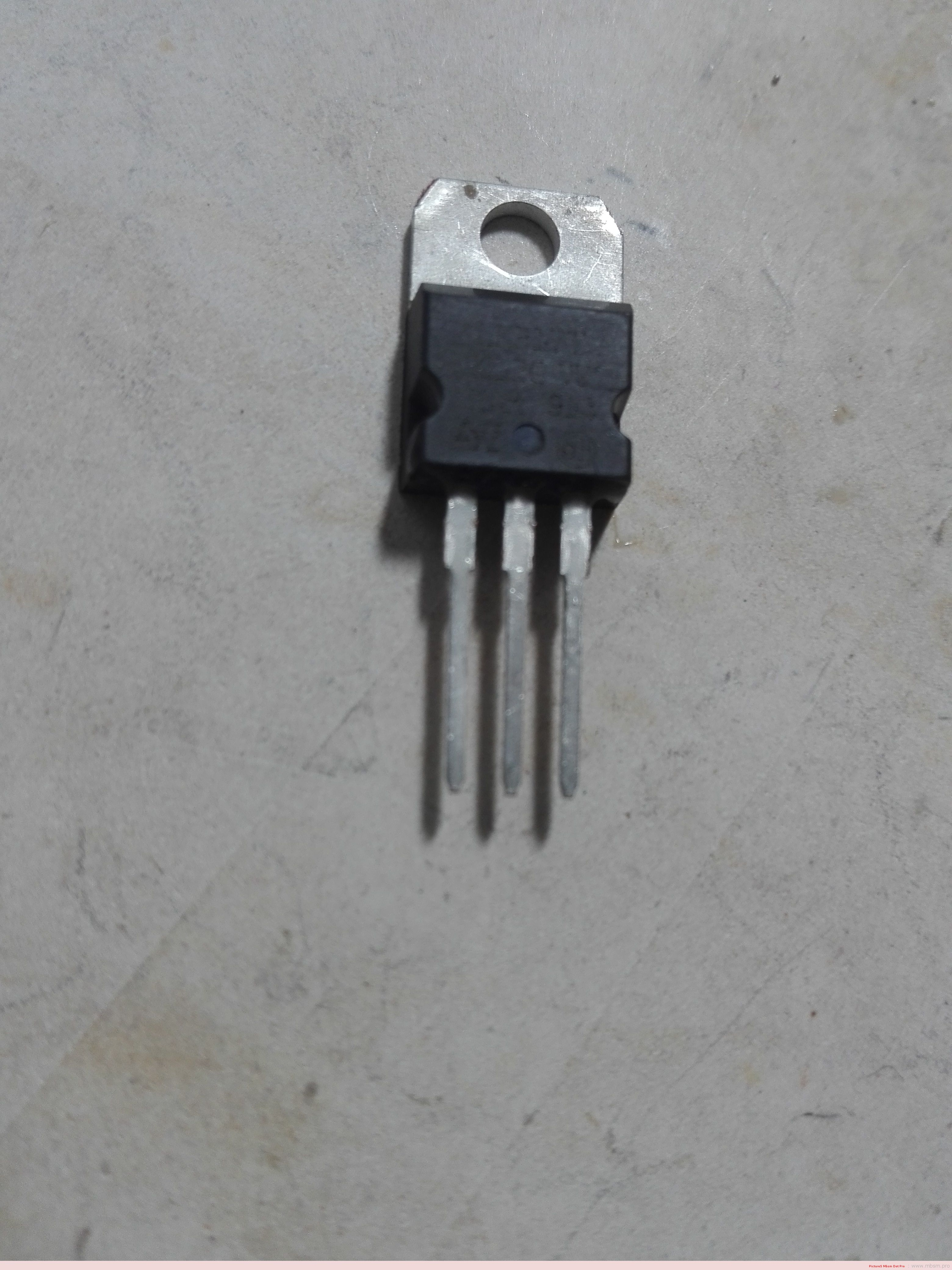##Mbsm.pro , LM317 ,Voltage Regulator Pin Outs, Simple test, voltage regulators ics , Voltage regulator

The LM317 is most commonly found in a TO220 package.  It only has three pins and we will be using all of them in this tutorial.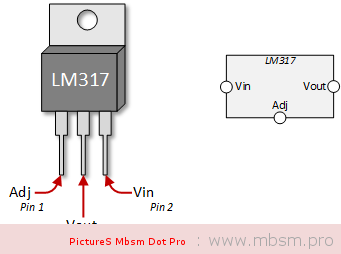# The LM317 Voltage Regulator for Current Control

The use of an LM317 as a constant current source comes right from the data sheet.     The schematic below shows how to configure the LM317 as a current regulator.   It is the value of R1 that you will be concerned about and that value is determined by the type of LED you are using.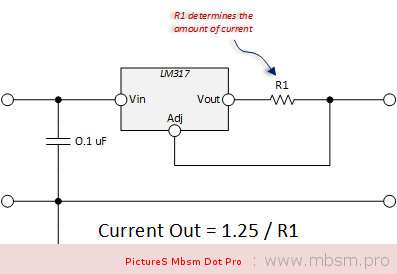The math is really simple. The factor of 1.25 also comes from the data sheet.

Let’s walk through an example:

1. Lets say you wanted to control to 300 mA.    You would determine that your optimum resistor is:
R1 = 1.25 / 0.300 = 4.17 Ohms
2. Next you’re going to poke around in your box of resistors to see what you’ve got.  You probably won’t find that 4.17 Ohm resistor, so you will want to try something close.   I had a 4.7 Ohm resistor.
3. Now you’re going to want to apply the formula to see what that gets you.
Current Out = 1.25 / 4.7 = 266 mA.
4. Finally, we need to do a sanity check of the power rating of the resistor.   Here we will use I2 x R to get the power dissipated by the resistor.
Power Dissipated by Resistor = 0.2662 x 4.7 = 0.332 Watts   (a half watt resistor will do the trick)